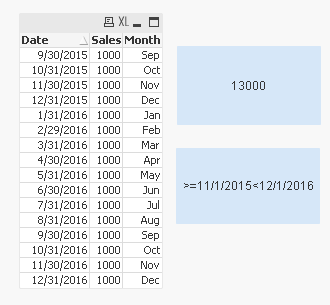# New to QlikView

Discussion board where members can get started with QlikView.

Announcements
Modernize Your QlikView Deployment webinar, Nov. 3rd. REGISTER
cancel
Showing results for
Did you mean:
HighlightedCreator III

## Calculating Rolling Periods using Set analysis

Hello, I'm trying to calculate rolling periods using set analysis. I have seen several posts related to calculating rolling periods, but most of them address issues using calendars, or the user is looking to calculating rolling "aggregated" periods, and not simply rolling periods.

Here's sample data with explanation:

GUAP:
Date, Sales
9/30/2015, 1000
10/31/2015, 1000
11/30/2015, 1000
12/31/2015, 1000
1/31/2016, 1000
2/29/2016, 1000
3/31/2016, 1000
4/30/2016, 1000
5/31/2016, 1000
6/30/2016, 1000
7/31/2016, 1000
8/31/2016, 1000
9/30/2016, 1000
10/31/2016, 1000
11/30/2016, 1000
12/31/2016, 1000
]
;

Final:
Date,
Sales,
Month(Date) AS Month

Resident GUAP;

Drop table GUAP;

I'm looking to use set analysis to return the last 12 months, excluding the current month, i.e. December 2016.

I've tried this:

=Sum({<Month={'>=\$(=Month(today(1))-13)<\$(=Month(today(1)))'}>} Sales)

and

=Sum({<Month={'>=\$(=Month(today(1))-13)<\$(=Month(today(1))-1)'}>} Sales)

The first formula returns all sales, i.e. 16,000

The second formula returns 12,000.

If this were calculating correctly, the formula would return 13,000, i.e. November 2015 - November 2016 Sales. Any help would be appreciated

1 Solution

Accepted Solutions
HighlightedMVP

Try this:

=Sum({<Date={"\$(='>=' & Date(MonthStart(Max(Date), -13)) & '<' & Date(MonthStart(Max(Date))))"}>} Sales)5 Replies
HighlightedMVP

Try this:

=Sum({<Date={"\$(='>=' & Date(MonthStart(Max(Date), -13)) & '<' & Date(MonthStart(Max(Date))))"}>} Sales)HighlightedCreator III

This is great Sunny. Thank you. It's correct based on the question I asked, but I think tweaking it a little bit will help others. Here I've just changed max(date) to today(1) which will always give a "true" 12 months rolling

.='>=' & Date(MonthStart(today(1), -12)) & '<' & Date(MonthStart(today(1)))

or in set analysis:

=Sum({<CanonicalDate={"\$(='>=' & Date(MonthStart(today(1), -12)) & '<' & Date(MonthStart(today(1))))"}>} Sales)

I do have one question though, how does qlikview know to evaluate '>=' and '<' since they are in quotes, I assumed QV would treat this as text that the user just wants inserted into their textbox, or whatever they're using. What if I really wanted my textbox, or whatever, to say 'X' &  '>' &  'Y'
Would QV look at this and try to actually evaluate X and Y as expressions?

HighlightedCreator III

Also, changing max(date) to today(1) will give the user to have this formula as a variable, and then make calls using multiple date fields, assuming they have >1 date field. In my case I have many (>5) date fields, so I have a canonical calendar where I can just use set analysis

=Sum(<{DateType = {'DateType'}, CanonicalDate = {\$(12MoRolling)}>} Sales)

HighlightedMVP

The dollar sign expansion around the expression evaluates it. So, essentially this -> ='>=' & Date(MonthStart(Max(Date), -13)) & '<' & Date(MonthStart(Max(Date))) becomes >=11/1/2015<12/1/2016

HighlightedContributor

Hello

I am working on a dataset where i have a column date (named DATE_NUM) that take the end of each month in "MM/DD/YYYY" format. I wanna make a sum on a contract quantity (named CONTR_QTY) with a rolling periods of the last 12month  but with others filters.

For example, fixed on the last 12 month AND on postpaid customer (telco sector). I have this : (but it's not working)

Sum({<{Date={"\$(='>=' & Date(MonthStart(Max(Date), -12)) & '<' & Date(MonthStart(Max(Date))))"},[Sheet1-1.TRF_MDL_PRE_POST_DESC]={"Postpaid"}>}CONTR_QTY)

Also if I need to divide this sum to have a ratio(example : the postpaid contracts/all the contract on this period) do I need to reuse the filter?

I am a bit lost, thank you for your help